# 15.9: Unsaturated Alcohols - Alkenols

$$\newcommand{\vecs}{\overset { \rightharpoonup} {\mathbf{#1}} }$$ $$\newcommand{\vecd}{\overset{-\!-\!\rightharpoonup}{\vphantom{a}\smash {#1}}}$$$$\newcommand{\id}{\mathrm{id}}$$ $$\newcommand{\Span}{\mathrm{span}}$$ $$\newcommand{\kernel}{\mathrm{null}\,}$$ $$\newcommand{\range}{\mathrm{range}\,}$$ $$\newcommand{\RealPart}{\mathrm{Re}}$$ $$\newcommand{\ImaginaryPart}{\mathrm{Im}}$$ $$\newcommand{\Argument}{\mathrm{Arg}}$$ $$\newcommand{\norm}{\| #1 \|}$$ $$\newcommand{\inner}{\langle #1, #2 \rangle}$$ $$\newcommand{\Span}{\mathrm{span}}$$ $$\newcommand{\id}{\mathrm{id}}$$ $$\newcommand{\Span}{\mathrm{span}}$$ $$\newcommand{\kernel}{\mathrm{null}\,}$$ $$\newcommand{\range}{\mathrm{range}\,}$$ $$\newcommand{\RealPart}{\mathrm{Re}}$$ $$\newcommand{\ImaginaryPart}{\mathrm{Im}}$$ $$\newcommand{\Argument}{\mathrm{Arg}}$$ $$\newcommand{\norm}{\| #1 \|}$$ $$\newcommand{\inner}{\langle #1, #2 \rangle}$$ $$\newcommand{\Span}{\mathrm{span}}$$

The simplest unsaturated alcohols, ethenol (vinyl alcohol), is unstable with respect to ethanal and has never been isolated (see Sections 10-5A and 13-5B):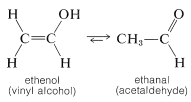Other simple unsaturated alkenols (enols) also rearrange to carbonyl compounds. However, ether and ester derivatives of enols are known and can be prepared by the addition of alcohols and carboxylic acids to alkynes. The esters are used to make many commercially important polymers (Chapter 29):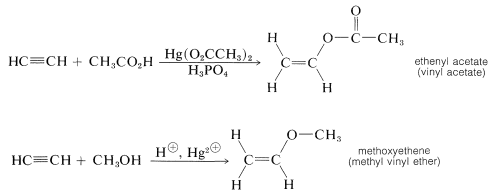The enol of 2-oxopropanoic acid (pyruvic acid) is of special biological interest because the phosphate ester of this compound is, like ATP (Section 15-5F), a reservoir of chemical energy that can be utilized by coupling its hydrolysis $$\left( \Delta G^0 = -13 \: \text{kcal} \right)$$ to thermodynamically less favorable reactions:In fact, the ester can be utilized to synthesize ATP from ADP; that is, it is a phosphorylating agent, and a more powerful one than ATP: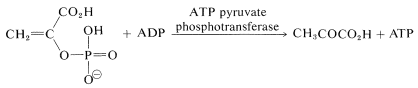## Acidity of Enols

Enols usually are unstable and are considerably more acidic than saturated alcohols. This means that the conjugate bases of the enols (the enolate anions) are more stable relative to the enols themselves than are alkoxide ions relative to alcohols. (Enolate anions are important reagents in the chemistry of carbonyl compounds and will be discussed in detail in Chapter 17.)The important factor here is delocalization of the negative charge on oxygen of enolate anions, as represented by the valence-bond structures $$14a$$ and $$14b$$: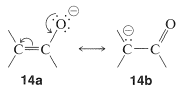Because acidity depends on the difference in energy of the acid and its conjugate base, we must be sure that the stabilization of the enolate anion by electron delocalization represented by $$14a$$ and $$14b$$ is greater than the analogous stabilization of the neutral enol represented by $$15a$$ and $$15b$$: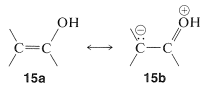The rules for evaluating valence-bond structures (Section 6-5B) tell us that the stabilization will be greatest when there are two or more nearly equivalent low-energy electron-pairing schemes. Inspection of $$14a$$ and $$14b$$ suggests that they will be more nearly equivalent than $$15a$$ and $$15b$$ because, although $$14b$$ and $$15b$$ have a negative charge on the carbon, in $$15b$$ the oxygen has a positive charge. Another way of putting it is that $$15b$$ represents an electron-pairing scheme with a charge separation, which intuitively is of higher energy than $$15a$$ with no charge separation. Structures corresponding to $$14b$$ and $$15b$$ are not possible for saturated alkanols or their anions, hence we can see that enols should be more acidic than alcohols.

Ascorbic acid (Vitamin C) is an example of a stable and quite acidic enol, or rather an enediol. It is a di-acid with p$$K_\text{a}$$ values of 4.17 and 11.57:Other important examples of stable enol-type compounds are the aromatic alcohols, or phenols. The $$K_\text{a}$$’s of these compounds are about $$10^{-10}$$, some $$10^8$$ times larger than the $$K_\text{a}$$’s for alcohols.The chemistry of these compounds, including their stability as enols, is discussed in Chapter 26.